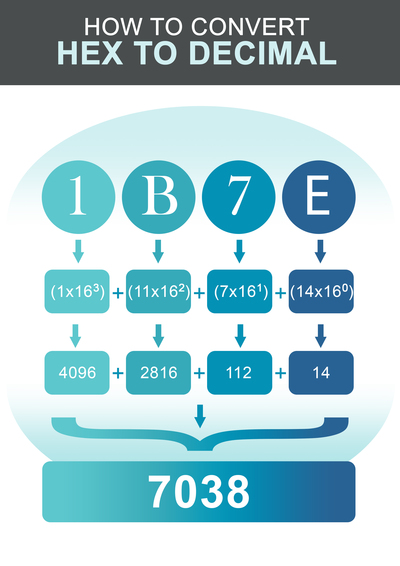Enter Hex Number:

## 如何将十六进制转换为十进制。\begin{array}{|c|c|c|c|c|c|c|} \hline \text{十六进制} & \text{1} & \text{B} & \text{7} & \text{E} \\ \hline \text{索引} & \text{3} & \text{2} & \text{1} & \text{0} \\ \hline \end{array}

\begin{array}{|c|c|c|c|c|c|c|} \hline \text{Hex code} & \text{A} & \text{B} & \text{C} & \text{D} & \text{E} & \text{F} \\ \hline \text{Dec Equiv.} & \text{10} & \text{11} & \text{12} & \text{13} & \text{14} & \text{15} \\ \hline \end{array}

\begin{array}{|c|c|c|c|c|c|c|} \hline \text{十六进制值的十进制} & \text{1} & \text{11} & \text{7} & \text{14} \\ \hline \text{索引} & \text{3} & \text{2} & \text{1} & \text{0} \\ \hline \end{array}

$$DEC = 4096 + 2816 + 112 + 14 = 7038$$

## 十进制数字系统。

$$245 = (2 \times 100) + (4 \times 10) + (5 \times 1)$$

## 为什么有些数字系统比其他系统更常见？

$$1010$$